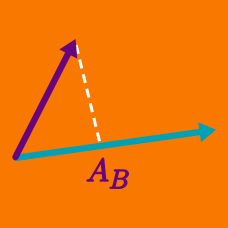Geometry

# Dot Product - Problem Solving

Consider the two vectors $\vec{a}=(3,4,5)$ and $\vec{b}=(1,1,1).$ What is the value of $t$ when the magnitude of $\vec{a}+t\vec{b}$ is minimum?

For what values of $b$ are the two vectors $(-4, b)$ and $(b, {b}^{2} )$ orthogonal?

If $\vec{a}=(3,4,1)$ and $\vec{b}=(2,4,5),$ what is the magnitude of the projection of $\vec{a}$ onto $\vec{b}?$

Consider a regular tetrahedron $OABC$ with edge length $1.$ $P$ is a point on $\overline{OA}$ such that $\overrightarrow{OA}\cdot\overrightarrow{PB}=\frac{1}{3}$ and $Q$ is a point on $\overline{PB}$ such that $\overrightarrow{PB}\cdot\overrightarrow{QC}=0.$ The vector $\overrightarrow{QC}$ can be expressed as $x\overrightarrow{OA}+y\overrightarrow{OB}+z\overrightarrow{OC}.$ Find the value of $31(x+y+z).$

Which of the following are correct where $\vec{a}$ and $\vec{b}$ are arbitrary vectors?

I. $\lvert\vec{a}\cdot\vec{b}\rvert\le\lvert\vec{a}\rvert\lvert\vec{b}\rvert$
II. $\lvert\vec{a}+\vec{b}\rvert\le\lvert\vec{a}\rvert+\lvert\vec{b}\rvert$
III. $\lvert\vec{a}-\vec{b}\rvert\le\lvert\vec{a}\rvert-\lvert\vec{b}\rvert$

×